# Test: Problems on Pythagoras Theorem

## 10 Questions MCQ Test NCERT Mathematics for CAT Preparation | Test: Problems on Pythagoras Theorem

Description
Attempt Test: Problems on Pythagoras Theorem | 10 questions in 10 minutes | Mock test for Class 10 preparation | Free important questions MCQ to study NCERT Mathematics for CAT Preparation for Class 10 Exam | Download free PDF with solutions
QUESTION: 1

### The monitors of computers are measured along the diagonal. What is the size of the largest monitor that can be placed in a space measuring 17″ x 21″?​

Solution:

Since monitors are measured along the diagonals this means we need to use pythagoras theorem for this right angled triangle.
H= P+ B2
=17 * 17 + 21 * 21
=289 + 441 = 730
H=27’’ (Approx)

QUESTION: 2

Solution:
QUESTION: 3

### Find the height of the given triangle if its base is 26 inches and the slant side is 15 inches long as shown below.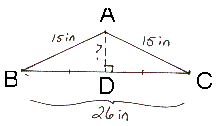​

Solution:

Height of the triangle is the altitude. SInce the triangle is an isosceles triangle its median is equal to its altitude . So this means that the altitude is perpendicular and bisects the opposite side .
So BD=DC=26/2=13
So in right angled Triangle,
Using Pythagoras theorem,
H= P+ B2
225 = P+ 169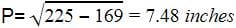QUESTION: 4

If the sum of the squares of a and b is the same as the square of c and if m < n, then for what value of k can the Pythagorean triples be generated with the following equations?
a = n2 + k , b = 2mn : c = m2 + n2

Solution:
QUESTION: 5

Find the total perimeter of the given figure.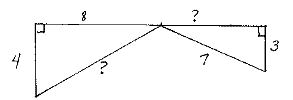Solution:
QUESTION: 6

What is the diagonal length of a TV screen whose dimensions are 80 cm x 60 cm?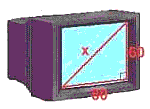Solution:
QUESTION: 7

If the sum of the length of the legs of a right triangle is 49 cm and the hypotenuse is 41 cm, find its shortest side.​

Solution:

Sum of the length of the legs of a right angle triangle is 49
So let one leg be x, other will be 49-x
Applying Pythagoras theorem,
H2=P2+B2
41*41=x2+(49-x)2
1681=x+ 2401 + x- 98x
2x2- 98x + 720 = 0
Dividing the equation by 2
x- 49x + 360 = 0
x- 9x - 40x + 360 = 0
x (x - 9) - 40 (x - 9) = 0
(x - 9)(x - 40) = 0
So x is either 9 or 40,
So the smaller side is 9 cm

QUESTION: 8

How far up a wall will an 11m ladder reach, if the foot of the ladder is 4m away from the wall?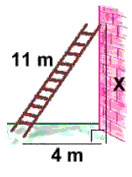Solution:

The height of wall will be the perpendicular for the right angle triangle. So applying pythagoras theorem
H2=P2+B2
11*11=P2+16
P=121-16=10.2 cm

QUESTION: 9

A wood frame for pouring concrete has an interior perimeter of 14 metres. Its length is one metre greater than its width. The frame is to be braced with twelve-gauge steel cross-wires. Assuming an extra half-metre of wire is used at either end of a cross-wire for anchoring, what length of wire should be cut for each brace?​

Solution:

Let width is x
Then length is x+1
Perimeter =x +x + x + 1 + x + 1 = 14
4 x= 12
x = 3
length = 4
Using Pythagoras theorem,
H2 = P+ B2
=9 + 16 = 25
H = 5cm
An extra half meter on either side will give the length of the wire as 5 + 1 = 6cm

QUESTION: 10

Anuj swims across a pool diagonally every day. The pool is 4 m wide, and it is 16 m diagonally across. What is the length of the pool?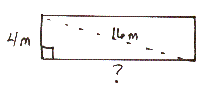Solution:Use Code STAYHOME200 and get INR 200 additional OFF Use Coupon Code